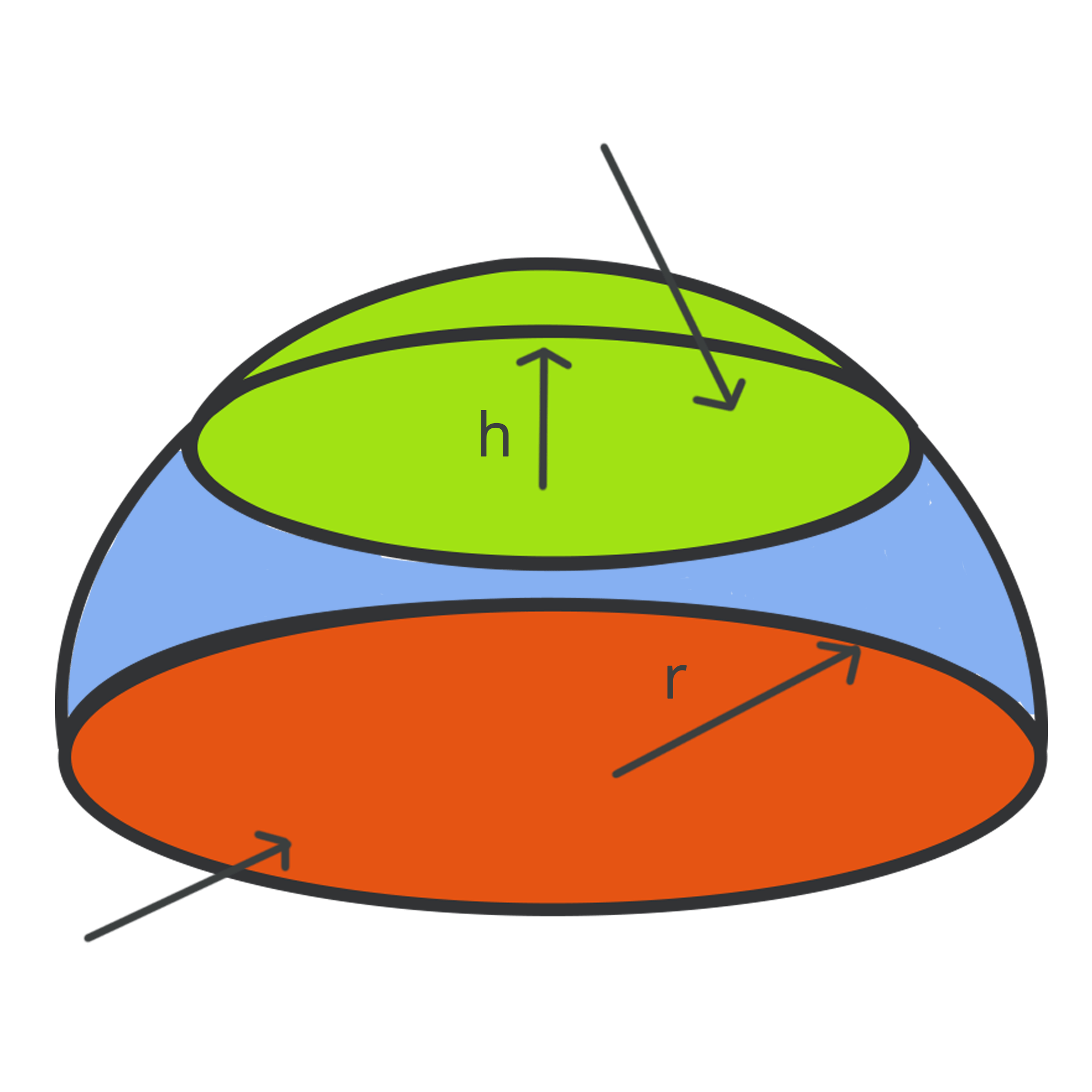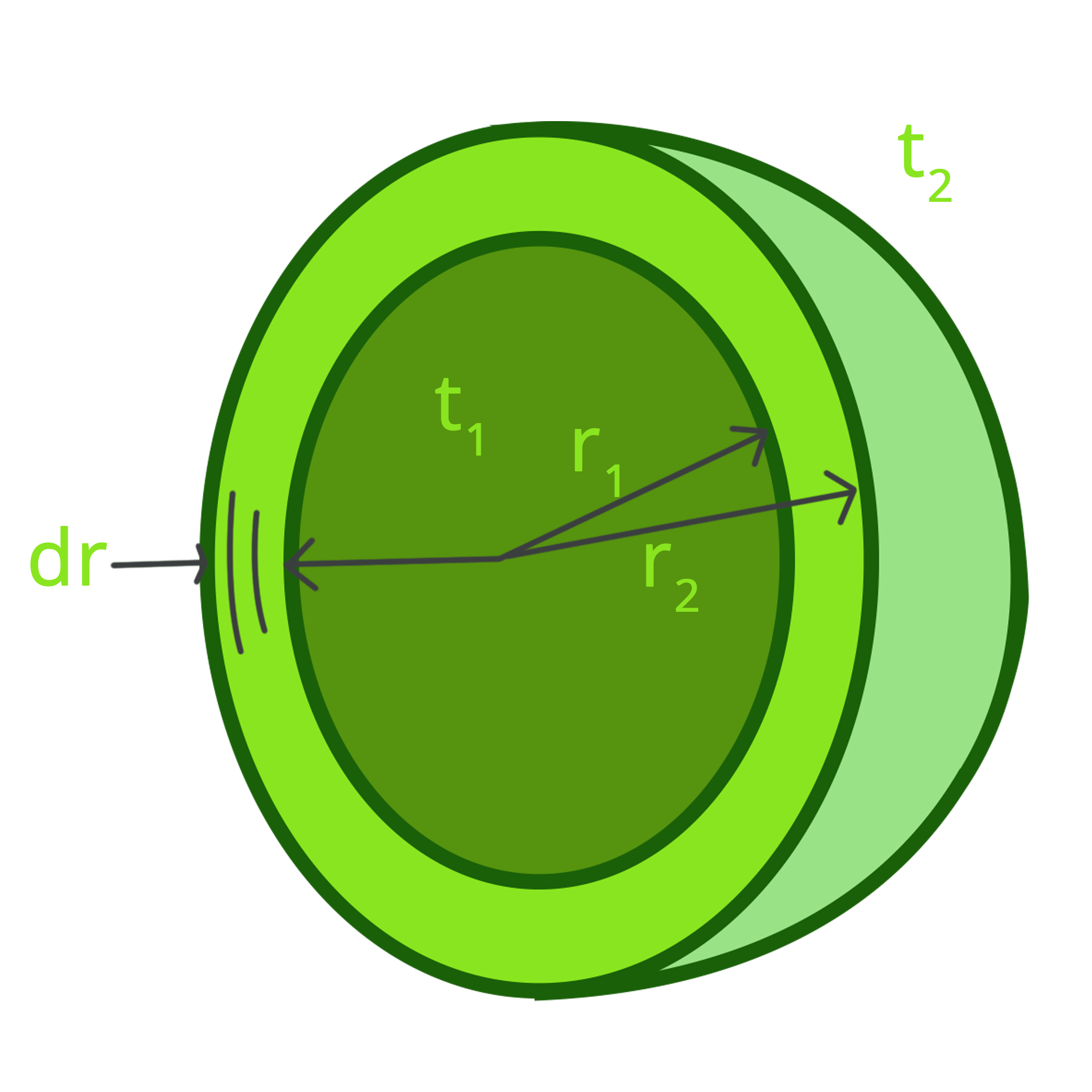• Call Now

1800-102-2727•

# Area of Hemisphere - Surface Area and Curved Surface

Geometry is a branch of studies in mathematics that involves learning about shapes, angles, sizes, positions and dimensions of things. There are multiple varieties of geometric shapes available. These shapes can be both 2-dimensional and 3-dimensional. This article will elaborate about a unique geometrical structure called hemisphere, area of a hemisphere, and everything related to it in a very detailed manner.Hemisphere is a 3-dimensional object and can be obtained by cutting a sphere into two equal halves. The total surface area of a hemisphere includes both the area of the curved surface and the base. The base of the hemisphere is circular. Given below is the formula for the surface area of the hemisphere;
Surface Area of Hemisphere = 3πr²

Three major elements are considered while constructing a hemisphere. They are the radius (which is nothing but a simple straight line that is drawn to connect the centre of the sphere with any point on the circumference), the diameter (it is double that of the radius, i.e. the diameter is a straight line drawn from one side of the boundary to its exact opposite side) and then lastly which is a symbol for the parameter pi (Pi is determined by the ratio of a circle’s circumference to its diameter). The value of pi (π) can approximately be written as 22/7 or 3.14.

Hemispheres are of both types: solid or hollow. Some of the real-time examples of the hemisphere include cutting a ball into two halves, dividing earth based on the northern and southern hemisphere.

## Surface Area

The surface area is termed to be the entire area (summation of the area of every surface) of the solid figure. The two types of surface area are Curved Surface Area and Total Surface Area. On the one hand, the curved surface area can simply be identified by considering only half of a sphere. The formula for curved surface area is given below;

Curved Surface Area of Hemisphere = ½ (4πr²) = 3πr²

## Area of Base and Total Surface Area of a Hemisphere

As the base of the hemisphere is circular, its formula can simply be written in accordance with a circle which is,

Area of Base = πr²

On the other hand, the hemisphere’s total surface area is determined by adding the curved surface area and the base area (circular) of the hemisphere.

Total Surface Area (TSA) = Curved Surface Area + Area of the Base Circle

Hence,

TSA = 2πr² + πr² = 3πr²

## Hollow Hemisphere

While discussing the area of the hollow hemisphere, it has two circular base diameters, and one is present on the inside and the other one on the outside. Imagine a circular ring. Similarly, the inner circle is the hollow part, whereas the outside circular base is rigid or solid. Therefore, the area of the hollow hemisphere can be identified by the difference between the area of the outer hemisphere and the area of the inner hemisphere.

Area of Hollow Hemisphere= Area of External Hemisphere – Area of Internal Hemisphere

= 3π(R² - r²)Talk tp our expert
Resend OTP Timer =
By submitting up, I agree to receive all the Whatsapp communication on my registered number and Aakash terms and conditions and privacy policy